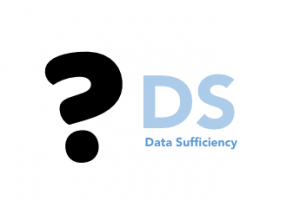true

Find the best tutors and institutes for GMAT Coaching

Find Best GMAT Coaching classes

No matching category found.

No matching Locality found.

Outside India?

Search in

GMAT Quantitative SectionThe arithmetic/mathematical segment of GMAT exam is precisely referred to as Quant section. In this section, 31 questions will be given and 62 minutes time to complete it. This part judges a test taker’s Quantitative reasoning, interpretation abilities and concluding skills from the available Graphical data.

Types of Questions in GMAT Quant section

It consists of two types of Questions:

• Problem Solving (PS)- This part mostly includes mathematics from class 10. So, if you have a clear concept of maths topics until 10th standard in mind, then, it won’t be very hard to crack.

• Data Sufficiency (DS)-  As the name suggests, this part of the Quantitative section is to see whether or not the given data is sufficient enough to solve a given

A. Statement (1) ALONE is sufficient, but statement (2) alone is not sufficient.

B. Statement (2) ALONE is sufficient, but statement (1) alone is not sufficient.

C. BOTH statements TOGETHER are sufficient, but NEITHER statement ALONE is sufficient.

D. EACH statement ALONE is sufficient.

E. Statements (1) and (2) TOGETHER are NOT sufficient.

Tips for Problem Solving Section in GMAT Quant Section

The three easy ways to begin with the Problem Solving section of GMAT exam are as follows:

a) Remember, all GMAT Problem solving maths can be solved in three steps. To achieve this, GMAT test takers just need to begin the sums in the correct way.

b) If it’s a geometry related quant question, make sure you redraw the pattern on your rough sheet for the accuracy of the calculation.

c) First, learn how to solve maths in precise methodologies and then, you can focus on the time limitation part.

d) Each GMAT exam has to solve 20-24 Problem-Solving maths questions during the GMAT exam.

e) At times, the Problem Solving section of the GMAT exam might have Roman Numbers. Do not get perplexed with them. Simply solve the sum and select the right roman number option from the given ones.

Sample Problem Solving questionData Sufficiency of Quant SectionThe five easy ways to begin with the Data Sufficiency section of GMAT exam are as follows:

1. Mug up the answer options
2. Read the Data Sufficiency question thoroughly
3. Pinpoint the query made in the Data Sufficiency problem
4. Consider each option individually and judge its chance of being the answer to the problem
5. Assumptions might work for GMAT Data Sufficiency quant problems

Sample Data Sufficiency question### Books for Quant Section (GMAT)

Here is a list of GMAT Quantitative Books to follow:

• GMAT Advanced Quant: 250+ Practice Problems & Bonus Online Resources (Manhattan Prep GMAT Strategy Guides)
• The Official Guide for GMAT Quantitative Review 2017 with Online Question Bank and Exclusive Video
• GMAT Foundations of Math: 900+ Practice Problems in Book and Online (Manhattan Prep GMAT Strategy Guides)
• Advanced GMAT Quant (Manhattan Prep GMAT Strategy Guides)
• Quantitative Aptitude for Competitive Examinations by R.S. Aggarwal

Noteworthy points for Quant Section (GMAT):

• Students are not allowed to use a calculator during GMAT Quant Section exam.
• Students can do mathematical rough work in an extra sheet while solving Quant section in GMAT exam.
• As each maths problems are solved and answered on the computer, students can only move forward to the next math problem. In GMAT quant section exam one cannot revert back technically on-screen.

https://www.urbanpro.com/assets/new-ui/sharing_job.png
UrbanPro

How can we Improve it?

Please enter your question below and we will send it to our tutor communities to answer it *

Name *

Enter a valid name.

Email *

Enter a valid email.

Email or Mobile Number: *

UrbanPro.com helps you to connect with the best GMAT Coaching classes in India. Post Your Requirement today and get connected.

## Also see

X

### Looking for GMAT Coaching Classes?

Find best tutors for GMAT Coaching Classes by posting a requirement.

• Post a learning requirement
• Get customized responses
• Compare and select the best### Looking for GMAT Coaching Classes?

Find best GMAT Coaching Classes in your locality on UrbanPro